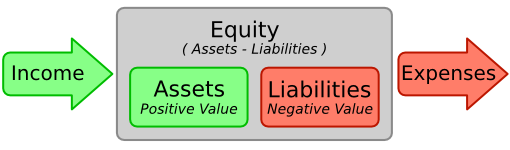## 2.1. Accounting Concepts

`GnuCash` is easy enough to use that you do not need to have a complete understanding of accounting principles to find it useful. However, you will find that some basic accounting knowledge will prove to be invaluable as `GnuCash` was designed using these principles as a template. It is highly recommended that you understand this section of the guide before proceeding.

### 2.1.1. The 5 Basic Accounts

Basic accounting rules group all finance related things into 5 fundamental types of accounts. That is, everything that accounting deals with can be placed into one of these 5 accounts:

Account types

Assets

Things you own

Liabilities

Things you owe

Equity

Overall net worth

Income

Increases the value of your accounts

Expenses

Decreases the value of your accounts

It is clear that it is possible to categorize your financial world into these 5 groups. For example, the cash in your bank account is an asset, your mortgage is a liability, your paycheck is income, and the cost of dinner last night is an expense.

### 2.1.2. The Accounting Equation

With the 5 basic accounts defined, what is the relationship between them? How does one type of account affect the others? Firstly, equity is defined by assets and liability. That is, your net worth is calculated by subtracting your liabilities from your assets:

Equation 2.1. The static accounting equation

Assets - Liabilities = Equity

Furthermore, you can increase your equity through income, and decrease equity through expenses. This makes sense of course, when you receive a paycheck you become richer and when you pay for dinner you become poorer. This is expressed mathematically in what is known as the Accounting Equation:

Equation 2.2. The (dynamic) accounting equation

Assets - Liabilities = Equity + (Income - Expenses)

This equation must always be balanced, a condition that can only be satisfied if you enter values to multiple accounts. For example: if you receive money in the form of income you must see an equal increase in your assets. As another example, you could have an increase in assets if you have a parallel increase in liabilities.

Figure 2.1. The basic accounts relationshipsA graphical view of the relationship between the 5 basic accounts. Net worth (equity) increases through income and decreases through expenses. The arrows represent the movement of value.

### 2.1.3. Debits and Credits

The use of debits and credits in accounting and their effect on accounts of different types is often confusing when first encountered in accounting. The accounting equation introduced above is the key to understanding which accounts types are debited or credited and when. First of all we need to rearrange the expanded form a little bit with Assets on the left hand side of the equal sign and transposing any account type with a negative sign to the other side to obtain:

Equation 2.3. The rearranged accounting equation

Assets + Expenses = Liabilities + Equity + Income

With the accounting equation in this form, the accounts on the left hand side of the equal sign are known as debit balance accounts in accounting practice, that is the normal positive balance for these accounts is increased by debit entries to accounts of these types. Conversely credit entries to accounts of these types will decrease the balance of accounts of these types.

Similarly, the account types on the right hand side of the equal sign are known as credit balance accounts, that is the normal positive balance for these account types is increased by credit entries to the accounts of these types. Again debit entries to accounts of these types will reduce the balance in the account.

Table 2.1. Summary of effect of debits (Dr) and credits (Cr) on the balance of accounts of the 5 account types

Account Type Effect on Account Balance
Debit (Dr) Credit (Cr)
Assets Increase Decrease
Expenses
Liabilities Decrease Increase
Equity
Income

### 2.1.4. Double Entry

The accounting equation is the very heart of a double entry accounting system. For every change in value of one account in the Accounting Equation, there must be a balancing change in another. This concept is known as the Principle of Balance, and is of fundamental importance for understanding `GnuCash` and other double entry accounting systems. When you work with `GnuCash`, you will always be concerned with at least 2 accounts, to keep the accounting equation balanced.

Balancing changes (or transfers of money) among accounts are done by debiting one account and simultaneously crediting another. Accounting debits and credits do not mean decrease and increase. Debits and credits each increase certain types of accounts and decrease others as described in the previous section. In asset and expense accounts, debits increase the balance and credits decrease the balance. In liability, equity and income accounts, credits increase the balance and debits decrease the balance.

In traditional double-entry accounting, the left column in the register is used for debits, while the right column is used for credits. Accountants record increases in asset and expense accounts on the debit (left) side, and they record increases in liability, income, and equity accounts on the credit (right) side. `GnuCash` follows this convention in the register.

NoteThis accounting terminology can be confusing to new users, which is why `GnuCash` allows you to use the common terms Deposit and Withdrawal. If you prefer the formal accounting terms, you can change the account register column headings to use them in the Accounts tab under Preferences (see the `GnuCash` Manual for more information on setting preferences).
WarningCommon use of the words debit and credit does not match how accountants use these words. In common use, credit generally has positive associations; in accounting, credit means affecting the right column of the ledger sheet of an account. This is associated with a decrease in asset and expense, but an increase of income, liability and equity accounts. For more details see https://en.wikipedia.org/wiki/Debits_and_credits.